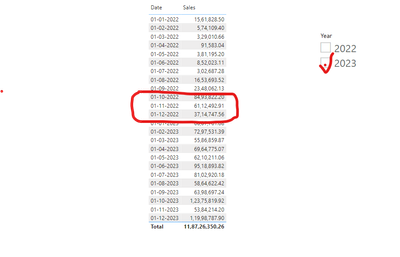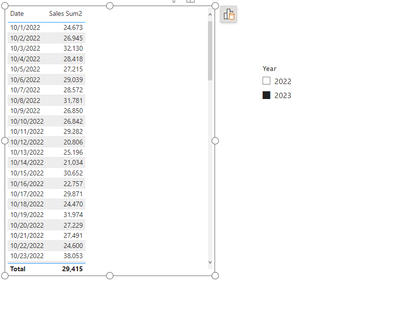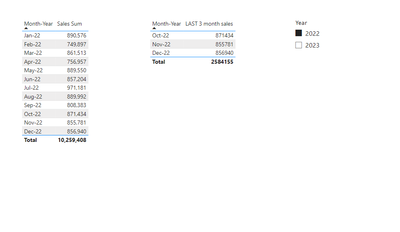cancel
Showing results for
Did you mean:

Fabric is Generally Available. Browse Fabric Presentations. Work towards your Fabric certification with the Cloud Skills Challenge.New Member

## I want show the Last year last 3 months sales when i select current year in slicer .

Hi Team,
I have finacials table.
I want to get the last year last 3 months sales value in my kart when i wan select the current year in slicer.
Example- I have data Starting from Jan 2022 to Dec 2023. When i select the 2023 in slicer ,i want see the sum of Oct,Nov,Dec value of 2022 in my kart.1 ACCEPTED SOLUTIONSuper User

i think i aswered wrong , you want if you press on 2023 to return 2022 last 3 months?

ok this is kinda tricky

Step 1 create a disconnected date table(date table without relationships)

step 2 add the dates you want to the table visual from the disconnected date tabel

step 3 create a filter measure that will show you your desire dates which are last 3 month of previous year

``````Filtering Dates =
VAR _m = CALCULATE(MAX('Table'[Month ID]),ALLEXCEPT('Table','Table'[Year]),'Table'[Year] = SELECTEDVALUE('Table'[Year])-1)
VAR weekstart = CALCULATE(MIN('Table'[Date]),REMOVEFILTERS('Table'),'Table'[Month ID] >= _m-2)
VAR weekend = CALCULATE(MAX('Table'[Date]),REMOVEFILTERS('Table'), AND('Table'[Month ID] = _m,'Table'[Month ID] >= _m-2))
VAR result = IF(AND(MIN('Disconnected Table'[Date]) >= weekstart, MAX('Disconnected Table'[Date]) <= weekend), 1, 0)
RETURN
result``````

insert this measure into your filter panel of your table vissual where you have dates from disconnected table and set it to is 1step 4

create a new sum measure

``Sales Sum2 = CALCULATE(SUM('Table'[Sales]),REMOVEFILTERS('Table'),'Table'[Date] = MAX('Disconnected Table'[Date]))``if you want to make it by month-year

chnage the date of disconnected table to month year

and change the sales measure to

``Sales Sum2 = CALCULATE(SUM('Table'[Sales]),REMOVEFILTERS('Table'),'Table'[Month ID] = MAX('Disconnected Table'[Month ID]))``if it helped please leave a kudos

2 REPLIES 2Super User

i think i aswered wrong , you want if you press on 2023 to return 2022 last 3 months?

ok this is kinda tricky

Step 1 create a disconnected date table(date table without relationships)

step 2 add the dates you want to the table visual from the disconnected date tabel

step 3 create a filter measure that will show you your desire dates which are last 3 month of previous year

``````Filtering Dates =
VAR _m = CALCULATE(MAX('Table'[Month ID]),ALLEXCEPT('Table','Table'[Year]),'Table'[Year] = SELECTEDVALUE('Table'[Year])-1)
VAR weekstart = CALCULATE(MIN('Table'[Date]),REMOVEFILTERS('Table'),'Table'[Month ID] >= _m-2)
VAR weekend = CALCULATE(MAX('Table'[Date]),REMOVEFILTERS('Table'), AND('Table'[Month ID] = _m,'Table'[Month ID] >= _m-2))
VAR result = IF(AND(MIN('Disconnected Table'[Date]) >= weekstart, MAX('Disconnected Table'[Date]) <= weekend), 1, 0)
RETURN
result``````

insert this measure into your filter panel of your table vissual where you have dates from disconnected table and set it to is 1step 4

create a new sum measure

``Sales Sum2 = CALCULATE(SUM('Table'[Sales]),REMOVEFILTERS('Table'),'Table'[Date] = MAX('Disconnected Table'[Date]))``if you want to make it by month-year

chnage the date of disconnected table to month year

and change the sales measure to

``Sales Sum2 = CALCULATE(SUM('Table'[Sales]),REMOVEFILTERS('Table'),'Table'[Month ID] = MAX('Disconnected Table'[Month ID]))``if it helped please leave a kudosSuper User

hello,

step 1 : add month ID in new column

``````Month ID =
VAR _min = YEAR(MIN('Table'[Date]))
RETURN
MONTH('Table'[Date]) + (YEAR('Table'[Date]) - _min) *12``````

step 2 make measures:

``Sales Sum = SUM('Table'[Sales])``

``````LAST 3 month sales =
VAR _m = CALCULATE(MAX('Table'[Month ID]),ALLEXCEPT('Table','Table'[Year]))
RETURN
CALCULATE(
[Sales Sum],
FILTER('Table',
'Table'[Month ID] >= _m -2))``````Announcements#### Power BI Monthly Update - November 2023

Check out the November 2023 Power BI update to learn about new features.#### Fabric Community News unified experience

Read the latest Fabric Community announcements, including updates on Power BI, Synapse, Data Factory and Data Activator.#### The largest Power BI and Fabric virtual conference

130+ sessions, 130+ speakers, Product managers, MVPs, and experts. All about Power BI and Fabric. Attend online or watch the recordings.Top Solution Authors
Top Kudoed Authors
Users online (4,502)